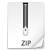# Variance-Covariance Matrix / Pearson Correlation Coefficient Matrix in VBA

Building a Variance-Covariance Matrix and a Pearson Correlation Coefficient Matrix in VBA

• Building a Variance-Covariance / Pearson Correlation Coefficient matrix in Excel can be cumbersome using formulas, the purpose of this model is to produce those matrices via VBA ;
• Users having Bloomberg can easily update time-series by specifying the ticker, other data sources can obviously be used by overriding series; as long as  the format is respected ;